# Data Structure questions for Computer Science students.

## What is Data Structure?

• Data structure is a group of data elements that are grouped together under one name.
• These data elements are called members. They can have different types and different lengths.
• Some of them store the data of the same type while others store different types of data.
• Data structures are used to store data in a computer in an organized fashion.

## What is ADT?

• ADT stands for Abstract Data Type.
• It is a mathematical model of a data structure.
• ADT describes a container which holds a finite number of objects where the objects may be associated through a given binary relationship.
• It is a logical description of how we view the data and the operations that are allowed without regard to how they will be implemented.
• It is concerned only with what the data is representing? And how it will eventually be constructed?
• It is a set of objects and operations, for example: list, insert, delete, search, sort.
Abstract Data Types (ADTs)

Following are the some basic ADTs of data structure
1. Stack
2. Queue

1. Stack
• It's a list where insert and remove take place only at the top.
• It performs LIFO(Last In First Out) operation.
Operations of Stack

Push: Insert element on top of stack.
Pop: Remove element from top of stack.
Top: Return element at top of stack.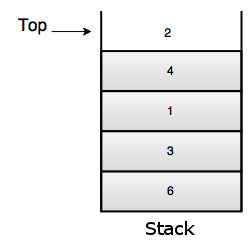2. Queue
• It's a list where insert takes place at the back, but remove takes place at the front.
• It performs FIFO(First In First Out) operation.
Operations of Queue

Enqueue: Insert element at the back of the queue.
Dequeue: Remove and return element from the front of the queue.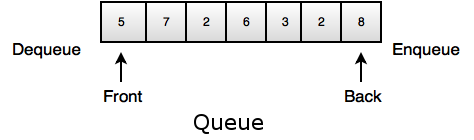• Linked list is a linear collection of data elements, called nodes pointing to the next node by means of pointer.
• Each node consists of its own data, address of the next node, forms and chain.
• It is used to create trees and graphs.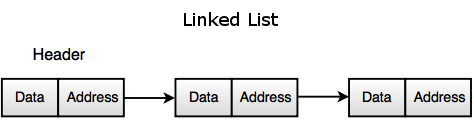• It is reusable and ensures robust data structure.
• It can be re-used at several places.
• It reduces coding efforts.
• Encapsulation ensures that data cannot be corrupted.
• It is based on principles of Object Oriented Programming (OOP) and Software Engineering (SE).

## What are the different applications of stack?

Some of the applications of stack are as follows:
• Function calls.
• Reversal of a string.
• Checking validity of an expression containing nested parenthesis.
• Conversion of an expression.
• a. Infix to Postfix
b. Infix to Prefix
c. Postfix to Infix
d. Prefix to Infix
• Evolution of an expression.
Representation of an Expression
InfixPrefixPostfix
A + B+ ABAB +
A + B * C+ A * BCABC * +
(A + B) * (C – D)* + AB – CDAB + CD – *

## Describe Stack operation.

Stack Operations

1. Push
• Push (inserts) the element in the stack. The location is specified by the pointer.
2. Pop
• Pulls (removes) the element out of the stack. The location is specified by the pointer.
3. Swap
• The two top most elements of the stack can be swapped.
4. Peek
• Returns the top element on the stack but does not remove it from the stack.
5. Rotate
• The topmost (n) items can be moved on the stack in a rotating fashion.
• A stack has a fixed location in the memory. When a data element is pushed in the stack, the pointer points to the current element.

## Which data structure is used to perform recursion?

• Stack is used to perform recursion in data structure.
• Its LIFO property helps to remember its caller. This caller helps to know the data which returns the function.
• System stack is used for storing the return addresses of the function calls.

## Can a stack be described as a pointer? Explain.

• Yes, a stack can be represented as a pointer.
• The reason is that, it has a head pointer which points to the top of the stack.
• The stack operations are performed using the head pointer. Hence, the stack can be described as a pointer.

## Is it possible to insert different types of element in a stack? How?

• Yes, it is possible to insert different types of elements in a stack.
• Elements in a stack can be inserted using the 'PUSH' operation.
• The 'PUSH' operation writes an element on the stack and moving the pointer.
• Different elements can be inserted into a stack. This is possible by implementing the union or a structure data type.
• It is efficient to use union rather than structure, as only one item’s memory is used at a time.

## Describe Queue operation.

Queue Operations

1. Push
• Inserts the element in the queue at the end.
2. Pop
• Removes the element out of the queue from the front.
3. Size
• Returns the size of the queue.
4. Front
• Returns the first element of the queue.
5. Empty
• To find if the queue is empty.

## What is a dequeue?

• A dequeue is a double-ended queue.
• It is an abstract data type similar to a simple queue.
• The elements here can be inserted or removed from either end.
• It allows to insert and delete from both sides means items can be added or deleted from the front and rear end.
• It is a sequence of data.
• The data can be added only at the front or at the end.
• The data can be removed only from the front or the end.
• The data can be examined only at the front and end.

## What are the difference between a Stack and a Queue?

StackQueue
Stack represents the collection of elements in Last in First Out (LIFO) order.Queue represents the collection of elements in First In First Out (FIFO) order.
Insertion and deletion operations are performed at same end.Insertion and deletion operations are performed at different end.
Only one pointer is used in stack which is called as TOP.Two pointers are used in queue which is called as FRONT and REAR.
There is no wastage of memory space.There is a wastage of memory space.
Example
Plate counter at marriage reception.
Example
Students standing in a line at fee counter.
An element is inserted at the top of the stack and removed from the top as well.An element is inserted at the end of a queue and is removed from the front.
It has a bounded capacity.It can be of bounded capacity, but is usually implemented without a specific capacity.
Stack operation includes testing null stacks, finding the top element in the stack, removal of top most element and adding elements on the top of the stack.Queue operation includes testing null queue, finding the next element, removal of elements and inserting the elements from the queue.

## Describe the following terms of a tree.

a) Level
b) Height
c) Degree

Let’s take an example to define the above term.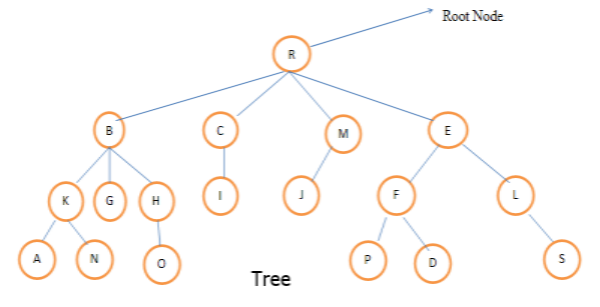a. Level:
• Level of any node is defined as the distance of that node from the root.
• The level of root node is always zero.
• Nodes B, C, M, E are at level 1.
• Nodes K, G, H, I, J, F, L are at level 2.
• Nodes A, N, O, P, D, S are at level 3.
b. Height:
• Height is also known as depth of the tree.
• Height of the root node is one.
• Height of a tree is equal to one more than the largest level number of the tree.
• The height of the above tree is 4.
c. Degree:
• The number of children of a node is called its degree.
• The degree of node R is 4, the degree of node B is 3, the degree of node S is 0.
• The degree of a tree is the maximum degree of the node of the tree.
• The degree of the above given tree is 4.

## Describe binary tree and its property.

• It is a special data structure which is used for main purpose that is data storage.
• In a binary tree, a node can have maximum two children, or in other words we can say a node can have 0, 1 or 2 children.
• It has a special condition that each node can have two children at maximum.
• It has benefits of both an ordered array and a linked list as search is as quick as in sorted array and insertion or deletion operation are as fast as in linked list.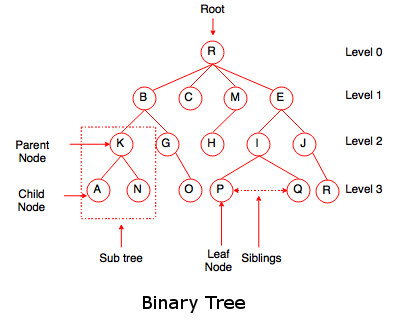Properties of binary tree
• The maximum number of nodes on any level i is 2i where i >= 0.
• The maximum number of nodes possible in a binary tree of height 'h' is 2h – 1.
• The minimum number of nodes possible in a binary tree of height 'h' is equal to h.
• If a binary tree contains 'n' nodes, then its maximum possible height is 'n' and minimum height possible is log2(n+1).
• If 'n' is the total no. of nodes and 'e' is the total no. of edges, then e = n - 1. The tree must be non-empty binary tree.
• If n0 is the number of nodes with no child and n2 is the number of nodes with 2 children, then n0 = n2 + 1.

## Describe full binary tree and complete binary tree.

Full Binary TreeComplete Binary Tree
If all the levels have maximum no. of nodes then it is a full binary tree.If all the levels have maximum no. of nodes except possibly that the last level, then it is a complete binary tree.
Height of full binary tree is (2h – 1) nodes, where 'h' is the height of the tree.The maximum no. of nodes in a complete binary tree is 2h – 1 and the maximum no. of nodes possible is (2h - 1), where 'h' is the height of the tree.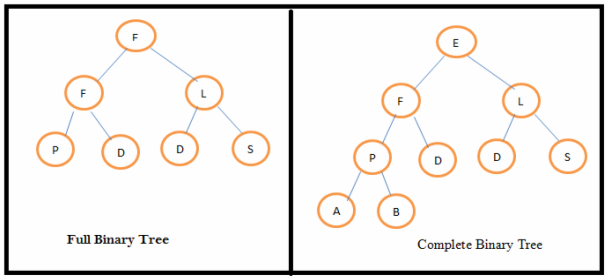## Explain Extended Binary Tree.

• A binary tree can be converted to an extended binary tree by adding special nodes to leaf nodes and nodes that have only one child.
• Extended binary tree is also called 2-tree.
•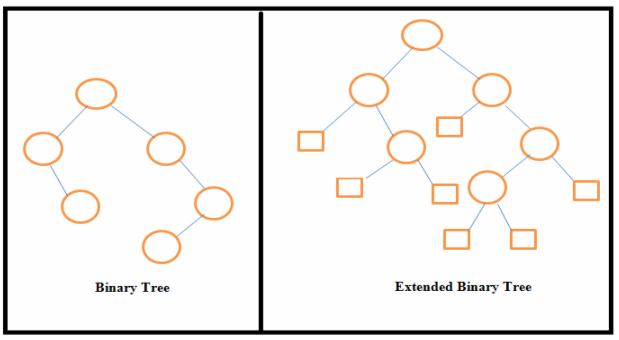• In the above figure, external nodes are shown by squares and internal nodes by circles.
• The extended binary tree is a strictly binary tree means each node has either 0 or 2 children.
• The path length of any node is the number of edges traversed from that node to the root node.
• Internal path length of a binary tree is the sum of path lengths of all internal nodes.
• External path length of a binary tree is the sum of path lengths of all external nodes.

## How does Threaded Binary Tree represented in Data Structure?

• Threaded binary tree consists of a node that is in the binary tree and having no left or right child.
• The child that comes in the end is also called as leaf node, and if there is no child present, then it will be represented by the null pointers.
• The space which is occupied by null entries that can be used to store valuable information about the node (consists of the child and the root node information).
• There is a special pointer that is used to point to the higher node in the tree also called as ancestor.
• These pointers are termed as threads and the whole representation is called as threaded binary tree.
• The binary tree can be represented in in-order, pre-order and post-order form.
• Every node in this type of tree doesn't have a right child.
• It does not allow the recursion of the tree.
The code that is used to show the implementation is:
struct NODE
{
struct NODE *leftchild;
int node_value;
struct NODE *rightchild;
}

## Describe AVL Tree or Height Balanced Binary Search Tree.

• An AVL Tree is a Binary Search Tree (BST), where the difference in the height of left and right subtrees of any node can be at most one.
• Balancing the binary search tree was introduced by Russian Mathematicians G. M. Adelson and E. M. Landis in 1962.
• The height balanced binary search tree is called AVL tree in their honor.

• Example: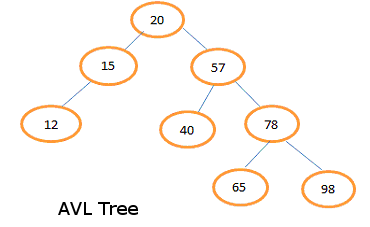• For the leaf node 12, 40, 65 and 98, left and right subtrees are empty so the difference of heights of their subtrees is 0.
• For node 20, height of left subtree is 2 and height of right subtree is 3 so the difference is 1.
• For node 15, height of left subtree is 1 and height of right subtree is 0 so the difference is 1.
• For node 57, height of left subtree is 1 and height of right subtree is 2 so the difference is 1.
• For node 78, height of left subtree is 1 and height of right subtree is 1 so the difference is 0.
• Each node of an AVL tree has a balance factor, which is defined as the difference between the heights of left subtree and right subtree of a node.
• Balance factor of a node = Height of its left subtree - Height of its right subtree
• In AVL tree, possible values for the balance factor of any node are: -1, 0, 1.

## You want to insert a new item in a binary search tree. How would you do it?

Let us assume that, you want to insert a unique item in a binary search tree.
• First of all, check if the tree is empty.
• If it is empty, you can insert the new item in the root node.
• If it is not empty, refer to the new item’s key.
• If the data to be entered is smaller than the root’s key, then insert it into the root’s left subtree.
• Else, insert it into the root’s right subtree.

## What is a recursive algorithm?

• Recursive algorithm is a method of simplification that divides the problem into sub-problems of the same nature.
• The result of one recursion is the input for the next recursion.
• The repletion is in the self-similar fashion.
• The algorithm calls itself with smaller input values and obtains the results by simply performing the operations on these smaller values.
• Generation of factorial, Fibonacci number series are the examples of recursive algorithms.

## Explain the following terms, a. Base Case b. Recursive Case c. Run-Time Stack d. Tail Recursion

a. Base Case
• A case in recursion, in which the answer is known when the termination for a recursive condition is to unwind back.
b. Recursive Case
• A case which returns the closer answer.
c. Run-time Stack
• Run time stack is used for saving the stack frame of a function when every recursion occurs.
• It consists of the return address and local variables.
d. Tail Recursion
• In tail recursion, a case where the function consists of a single recursive call.
• It is the last statement to be executed. It can be replaced by iteration.

## Explain Linked List.

• A linked list is a linear arrangement of data.
• It allows the programmer to insert data anywhere within the list.
• The pointer of the list always points to the first node and can be moved programmatically to insert, delete or update any data.
• Each node in the list contains a data value and the address or a reference to the adjoining node.
• A linked list is a dynamic data structure.
• It consists of a sequence of data elements and a reference to the next record in the sequence.

## Explain the types of linked lists.

The types of linked lists are:
1. Singly linked list
2. Doubly linked list
3. Circular linked list

1. Singly linked list
It has only head part and corresponding references to the next nodes.

2. Doubly linked list
A linked list which has both head and tail parts, thus allowing the traversal in bi-directional fashion. Except the first node, the head node refers to the previous node.

3. Circular linked list
A linked list whose last node has a reference to the first node.

## Describe the steps that how to insert a data into a Singly Linked List?

Steps to insert a data into a Singly Linked List

A. Insert in Middle

Data: 1, 2, 3, 5

1. Locate the node after which a new node (say 4) needs to be inserted (say after 3).
2. Create the new node i.e. 4.
3. Point the new node 4 to 5. (new_node → next = node → next(5))
4. Point the node 3 to node 4. (node → next =new_node)

B. Insert in the Beginning

Data: 2, 3, 5

1. Locate the first node in the list (2).
2. Point the new node (say 1) to the first node. (new_node → next = first)

C. Insert in the End

Data: 2, 3, 5

1. Locate the last node in the list (5).
2. Point the last node (5) to the new node (say 6). (node → next = new_node)

## Explain how to reverse Singly Linked List?

Reverse a Singly Linked List by using iteration.
• First, set a pointer (*current) to point to the first node i.e. current = base.
• Move ahead until current != null (till the end).
• Set another pointer (*next) to point to the next node i.e. next = current → next.
• Store reference of (*next) in a temporary variable (*result) i.e current → next = result.
• Swap the result value with current i.e. result = current.
• And now swap the current value with next. i.e. current = next.
• Return result and repeat from step 2.
• A linked list can also be reversed using recursion which eliminates the use of a temporary variable.

## How would you sort a linked list?

Following are the steps for sorting the linked list:

Step 1
Compare the current node in the unsorted list with every element in the rest of the list. If the current element is more than any other element go to Step 2 otherwise go to Step 3.

Step 2
Position the element with higher value after the position of the current element. Compare the next element. Go to Step 1 if an element exists, else stop the process.

Step 3
If the list is already in sorted order, insert the current node at the end of the list. Compare the next element, if any, and go to step 1 or quit.

## What is the use of space complexity and time complexity?

Space complexity
• The space complexity defines the storage capacity for the input data.
• It defines the amount of memory that is required to run a program to completion.
• The complexity like this depends on the size of the input data and the function that is used for the input size 'n'.
Time complexity
• The time complexity deals with the amount of time required by a program to complete the whole process of execution.
• It allows creating optimized code and allowing user to calculate it before writing their own functions.
• The time complexity can be made such that a program can be optimized on the basis of the chosen method.

## What is linear search?

• Linear search is the simplest form of search.
• It searches for the element sequentially starting from the first element.
• This search has a disadvantage if the element is located at the end. Advantage lies in the simplicity of the search.
• It is most useful when the elements are arranged in a random order.

## What is Binary Search and Fibonacci Search?

Binary Search
• Binary search is the process of locating an element in a sorted list.
• It is used to find an element of a sorted list only.
• The search starts by dividing the list into two parts.
• The algorithm compares the median value.
• If the search element is less than the median value, the top list only will be searched, after finding the middle element of that list.
• The process continues until the element is found or the search in the top list is completed.
• The same process is continued in the bottom list, until the element is found or the search in the bottom list is completed.
• If an element is found that must be the median value.
Fibonacci Search
• Fibonacci search is a process of searching a sorted array by utilizing divide and conquer algorithm.
• It is used to search an element of a sorted array with the help of Fibonacci numbers.
• Fibonacci number is subtracted from the index thereby reducing the size of the list.
• Fibonacci search examines the location whose addresses have a lower dispersion.
• When the search element has non-uniform access memory storage, the Fibonacci search algorithm reduces the average time needed for accessing a storage location.

## What is Bubble Sort and Quick sort?

Bubble Sort
• Bubble sort is the simplest sorting algorithm.
• It involves the sorting list in a repetitive fashion.
• It compares two adjacent elements in the list, and swaps them if they are not in the designated order.
• It continues until there are no swaps needed.
• This is the signal for the list that is sorted.
• It is also called as comparison sort as it uses comparisons.
Quick Sort
• Quick sort is the best sorting algorithm which implements the ‘divide and conquer’ concept.
• It first divides the list into two parts by picking an element a ’pivot’.
• It arranges the elements those are smaller than the pivot into one sub list and the elements those are greater than pivot into one sub list by keeping the pivot in its original place.

## Explain Merge Sort Algorithm?

Merge Sort
• Merge Sort is a comparison based sorting algorithm.
• The input order is preserved in the sorted output.
Merge Sort algorithm steps are as follows:

Step 1
The length of the list is 0 or 1, and then it is considered as sorted.

Step 2
Otherwise, divide the unsorted list into two lists, each about half the size.

Step 3
Sort each sub list recursively. Implement the Step 2 until the two sub lists are sorted.

Step 4
As a final step, combine (merge) both the lists back into one sorted list.

## Define a Linear and Non Linear data structure.

Linear data structure
• Linked list is an example of linear data storage or structure.
• Linked list stores data in an organized a linear fashion.
• They store data in the form of a list.
• It traverses the data elements sequentially, in which only one data element can directly be reached.
Non Linear data structure
• Tree data structure is an example of a non linear data structure.
• A tree has one node called as root node that is the starting point that holds data and links to other nodes.
• Every data item is attached to several other data items in a way that is specific for reflecting relationships.